# n 阶贝塞尔曲线计算公式实现

### n 阶贝塞尔曲线计算公式实现

#### 1. 效果参考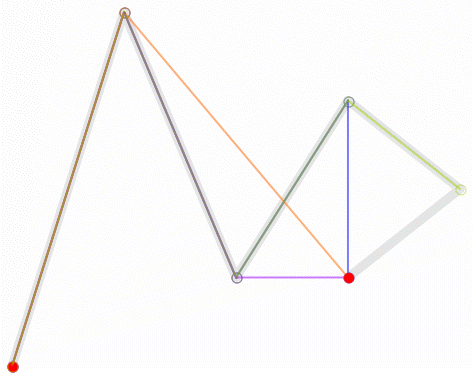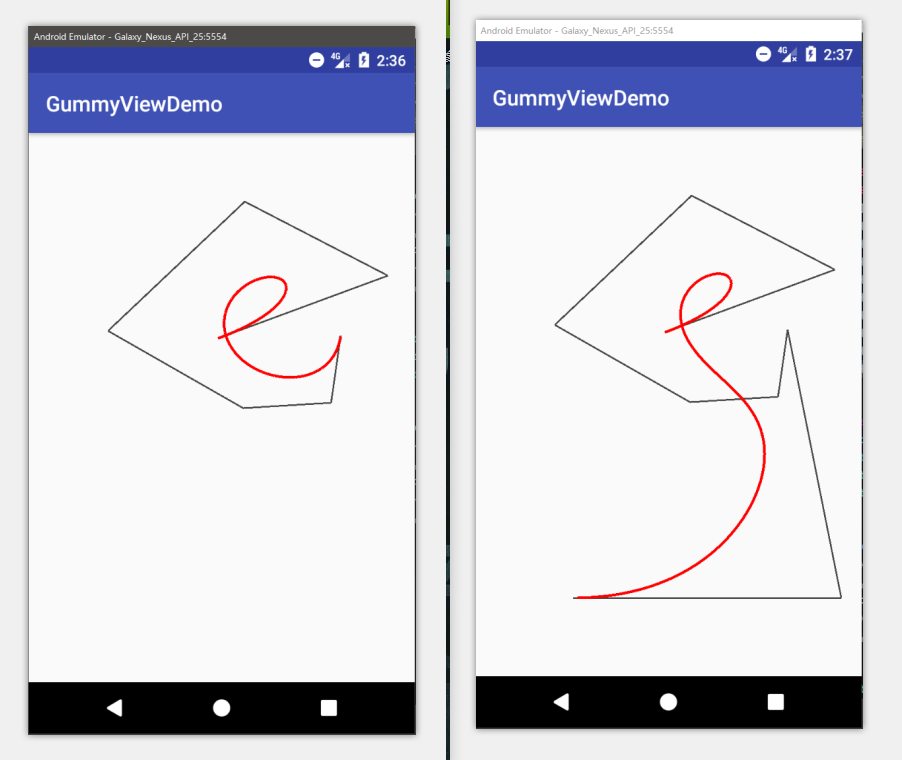#### 2. 思路解析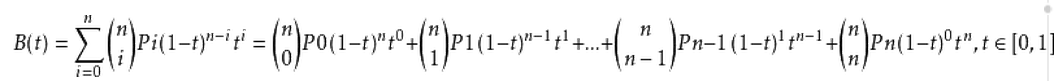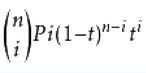• 从 i 递增到 n 的常数部分
• Pi 坐标部分
• (1 - t)^(n - i)
• t^i
可以看出这四部分都与 i 的值相关，此外 t 值的计算方式为：i/(n+1)
##### 如果直接从上面的公式上找规律比较抽象，那就从具体的例子中找规律吧：

• N = 3: P = (1-t)^2*P0 + 2*(1-t)*t*P1 + t^2*P2
• N = 4: P = (1-t)^3*P0 + 3*(1-t)^2*t*P1 + 3(1-t)*t^2*P2 + t^3*P3
• N = 5: P = (1-t)^4*P0 + 4*(1-t)^3*t*P1 + 6(1-t)^2*t^2*P2 + 4*(1-t)*t^3*P3 + t^4*P4

a * (1 - t)^b * t^c * Pn;

• N = 3:   1,2,0   2,1,1   1,0,2
a: 1 2 1
b: 2 1 0
c: 0 1 2
• N = 4:   1,3,0   3,2,1   3,1,2   1,0,3
a: 1 3 3 1
b: 3 2 1 0
c: 0 1 2 3
• N = 5:   1,4,0   4,3,1   6,2,2   4,1,3   1,0,4
a: 1 4 6 4 1
b: 4 3 2 1 0
c: 0 1 2 3 4

• b: (N - 1) 递减到 0 (b 为 1-t 的幂)
• c: 0 递增到 (N - 1) (c 为 t 的幂)
• a: 在 N 分别为 1,2,3,4,5 时将其值用如下形式表示：
N=1:———1
N=2:——–1  1
N=3:——1  2  1
N=4:—–1  3  3  1
N=5:—1  4  6  4  1
a 值的改变规则为： 杨辉三角

#### 3. 使用 java 来实现

float[] p0 = {3, 8};
float[] p1 = {4, 3};
float[] p2 = {2, 7};
float[][] result = new float;
for (int i = 0; i < 10; i++) {
float t = i / 10;
result[i] = (float) (1 * Math.pow(1 - t, 2) * Math.pow(t, 0) * p0 + 2 * Math.pow(1 - t, 1) * Math.pow(t, 1) * p1 + 1 * Math.pow(1 - t, 0) * Math.pow(t, 2) * p2);
result[i] = (float) (1 * Math.pow(1 - t, 2) * Math.pow(t, 0) * p0 + 2 * Math.pow(1 - t, 1) * Math.pow(t, 1) * p1 + 1 * Math.pow(1 - t, 0) * Math.pow(t, 2) * p2);
}

     /**
* @param poss      贝塞尔曲线控制点坐标
* @param precision 精度，需要计算的该条贝塞尔曲线上的点的数目
* @return 该条贝塞尔曲线上的点（二维坐标）
*/
public float[][] calculate(float[][] poss, int precision) {

//维度，坐标轴数（二维坐标，三维坐标...）
int dimersion = poss.length;

//贝塞尔曲线控制点数（阶数）
int number = poss.length;

//控制点数不小于 2 ，至少为二维坐标系
if (number < 2 || dimersion < 2)
return null;

float[][] result = new float[precision][dimersion];

//计算杨辉三角
int[] mi = new int[number];
mi = mi = 1;
for (int i = 3; i <= number; i++) {

int[] t = new int[i - 1];
for (int j = 0; j < t.length; j++) {
t[j] = mi[j];
}

mi = mi[i - 1] = 1;
for (int j = 0; j < i - 2; j++) {
mi[j + 1] = t[j] + t[j + 1];
}
}

//计算坐标点
for (int i = 0; i < precision; i++) {
float t = (float) i / precision;
for (int j = 0; j < dimersion; j++) {
float temp = 0.0f;
for (int k = 0; k < number; k++) {
temp += Math.pow(1 - t, number - k - 1) * poss[k][j] * Math.pow(t, k) * mi[k];
}
result[i][j] = temp;
}
}

return result;
}

........
// calculate 方法在 BezierImpl 中实现
private BezierImpl bezier = new BezierImpl();
private Paint paint = new Paint();
float[][] poss = {
{353.0f, 383.0f},
{670.0f, 266.0f},
{403.0f, 128.0f},
{148.0f, 369.0f},
{400.0f, 513.0f},
{564.0f, 503.0f},
{582.0f, 378.0f},
{682.0f, 878.0f},
{182.0f, 878.0f}
};

@Override
protected void onDraw(Canvas canvas) {
float x0, y0, x, y;
paint.setColor(Color.DKGRAY);
paint.setStrokeWidth(3.0f);
x0 = poss;
y0 = poss;
for (int i = 1; i < poss.length; i++) {
x = poss[i];
y = poss[i];
canvas.drawLine(x0, y0, x, y, paint);
x0 = x;
y0 = y;
}

paint.setColor(Color.RED);
paint.setStrokeWidth(5.0f);
float[][] po = bezier.calculate(poss, 500);
x0 = po;
y0 = po;
for (int i = 1; i < 500; i++) {
x = po[i];
y = po[i];
canvas.drawLine(x0, y0, x, y, paint);
x0 = x;
y0 = y;
}
}
...........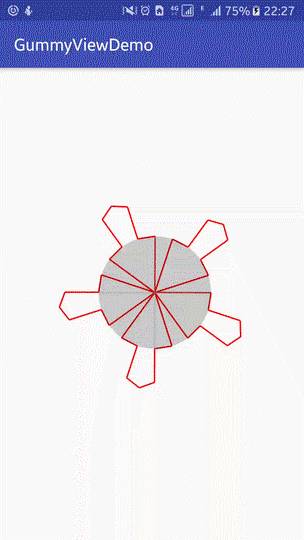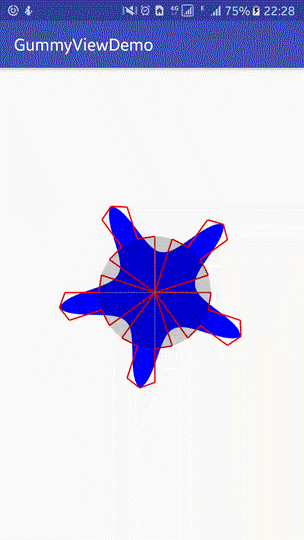END

06-10460603-05251
12-201279
05-265917
03-25869
07-132万+
01-091万+
08-035336
09-122084
03-141053
09-011万+
08-131804
08-18274
08-089535
02-18698
10-139681
09-061万+
12-28797点击重新获取扫码支付余额充值